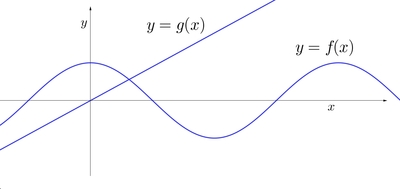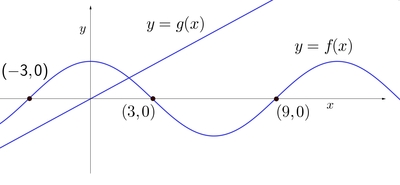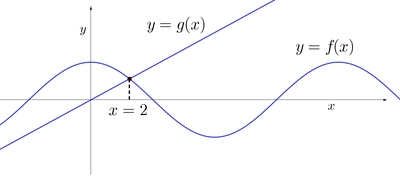# Interpreting the graph

Alignments to Content Standards: F-IF.A

Use the graph (for example, by marking specific points) to illustrate the statements in (a)–(d). If possible, label the coordinates of any points you draw.1. $f(0) = 2$
2. $f(−3)=f(3)=f(9)=0$
3. $f(2) = g(2)$
4. $g(x)>f(x)$ for $x>2$

## IM Commentary

The purpose of this task is to help students learn to read information about a function from its graph, by asking them to show the part of the graph that exhibits a certain property of the function. The task could be used to further instruction on understanding functions, or as an assessment tool with the caveat that it requires some amount of creativity to decide how to best illustrate some of the statements.

## Solution

1.2.3.4.